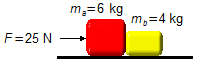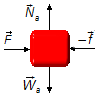Solved Problem on DynamicsPortuguêsEnglish

Two bodies of masses mA = 6 kg and mB = 4 kg are on a frictionless horizontal surface. A horizontal force of constant magnitude equal to 25 N is applied to push the two bodies. Find the acceleration acquired by the set and the magnitude of the contact force between the bodies.Problem diagram

We use a frame of reference oriented to the right in the same direction of the applied force, this produces an acceleration a on the system.

Problem data
• mass of body A:    mA = 6 kg;
• mass of body B:    mB = 4 kg;
• force applied to the system:    F = 25 N.figure 1
Solution

Drawing a free-body diagram for each body, we have for the body A
Horizontal direction
• $$\vec{F}$$ : force applied to the body;
• $$-\vec{f}$$ : normal force of body B on A.
Vertical direction
• $$\vec{N}_{\text{A}}$$ : normal force of the surface on the body;
• $$\vec{P}_{\text{A}}$$ : weight of body A.figure 2
In the vertical, direction there is no motion, normal force and weight cancel out.
In the horizontal, direction using Newton's Second Law
$\bbox[#99CCFF,10px] {\vec{F}=m\vec{a}}$
$F-f=m_{\text{A}}a \tag{I}$
For body B, we have

Horizontal direction
• $$\vec{{f}}$$: force of body A on B.
Vertical direction
• $${\vec{{N}}}_{\text{B}}$$: normal force of the surface on the body;
• $${\vec{{P}}}_{\text{B}}$$: weight of body B.figure 3
In the vertical direction, there is no motion, normal force and weight cancel out.
In the horizontal direction applying Newton's Second Law
${f=m_{\text{B}}a} \tag{II}$
Equations (I) and (II) can be written as a system of linear equations with two variables (f and a)
$\left\{ \begin{array}{rr} F-f&=m_{\text{A}}a\\ f&=m_{\text{B}}a \end{array} \right.$
substituting (II) into (I) we have the acceleration
$F-m_{\text{B}}a=m_{\text{A}}a\\ F=m_{\text{A}}a+m_{\text{B}}a$
factoring the acceleration
$F=a\;(\;m_{\text{A}}+m_{\text{B}}\;)\\ a=\frac{F}{m_{\text{A}}+m_{\text{B}}}\\ a=\frac{25}{6+4}\\ a=\frac{25}{10}$
$\bbox[#FFCCCC,10px] {a=2.5\;\text{m/s}^{2}}$

Note: Since the two bodies form a set subjected to the same force, both have the same acceleration, the system behaves as if it were a single body with total mass given by the sum of the masses of the two bodies A and B.

Substituting the acceleration found in expression (II) we have the force of contact between the bodies
$f=4\times2.5$
$\bbox[#FFCCCC,10px] {f=10\;\text{N}}$

Note: In the same way we could substitute the acceleration in the expression (I) to obtain the contact force, in this case:
$25-f=6\times2.5\Rightarrow 25-f=15\Rightarrow f=25-15\Rightarrow f=10\;\text{N}$